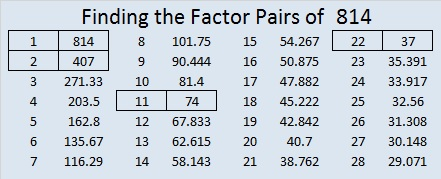# 814 and Level 6

814 is even, so it is divisible by 2. What else is it divisible by? Let’s apply 11’s divisibility rule:

• 8 – 1 + 4 = 11, so 814 can also be evenly divided by 11. (If the sum of every other digit minus the sum of the missed digits is divisible by 11, then the number is divisible by 11.)

This Level 6 Puzzle is a doozie, but I assure you that it CAN BE be solved using logic without guessing and checking!Print the puzzles or type the solution on this excel file: 10-factors 807-814

Now here’s a little bit more about the number 814:

• 814 is a composite number.
• Prime factorization: 814 = 2 x 11 x 37
• The exponents in the prime factorization are 1, 1, and 1. Adding one to each and multiplying we get (1 + 1)(1 + 1)(1 + 1) = 2 x 2 x 2 = 8. Therefore 814 has exactly 8 factors.
• Factors of 814: 1, 2, 11, 22, 37, 74, 407, 814
• Factor pairs: 814 = 1 x 814, 2 x 407, 11 x 74, or 22 x 37
• 814 has no square factors that allow its square root to be simplified. √814 ≈ 28.530685Because 37 is one of its factors, 814 is the hypotenuse of a Pythagorean triple:

• 264-770-814 which is 22 times (12-35-37)

814 is also repddigit MM in BASE 36 (M is 22 base 10) because 22(36) + 22(1) = 22(36 + 1) = 22 × 37 = 814

Here are some initial logical notes to solve Find the Factors Puzzle #814:

• 10, 8, 4, and 6 must use all the 1’s and 2’s, so clue 4 cannot use both 2’s and must use 1 & 4.
• The 8’s must be used by 72 and one of the 40’s. That 40 will also use a 5.
• 63 & 72 will use both 9’s so 18 must use a 3, as does 21.
• That means 30 can’t be 3 x 10 and must use a 5.
• Thus a 40 and 30 will use both 5’s so clue 10 can’t use a 5 and must use 1 & 10In case there is still any confusion, here’s where the factors went:This site uses Akismet to reduce spam. Learn how your comment data is processed.